# The diameter

The diameter of a circle is 4 feet. What is the circle's circumference?

o =  12.5664 ft

### Step-by-step explanation: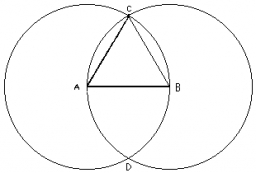Did you find an error or inaccuracy? Feel free to write us. Thank you!#### You need to know the following knowledge to solve this word math problem:

We encourage you to watch this tutorial video on this math problem:

## Related math problems and questions:

• Circle simpleCircumference of a circle is 6.28. What is the area of the circle?
• Square and circle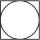Into square is an inscribed circle with diameter 10 cm. What is the difference between circumference square and circle?
• Circle - simpleThe circumference of a circle is 198 mm. How long in mm is its diameter?
• Diameter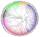The diameter of a circular plot is 14 dm. Find the circumference and area.
• Rectangle areaWhat is the area of a rectangle that has a length of 4 feet and a width of 4/10 feet?
• Central angleWhat is the length of the arc of a circle with a diameter of 46 cm, which belongs to a central angle of 30°?
• Diameter to areaFind the area of a circle whose diameter is 26cm.
• CircleHow big is an area of a circle if its circumference is 51.2 cm?
• Velocipede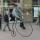The front wheel of velocipede from the year 1880 had a diameter 1.8 m. Suppose the front wheel turned again one, then the rear wheel 6 times. What was the diameter of the rear wheel?
• Perimeter of squareThe square has a circumference 17cm. What is its area?
• Four circles1) Calculate the circle radius if its area is 400 cm square 2) Calculate the radius of the circle whose circumference is 400 cm. 3) Calculate circle circumference if its area is 400 cm square 4) Calculate the circle's area if perimeter 400 cm.
• The perimeter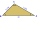The triangle has one side 5 cm long and the another 11 cm long. What can be the smallest and what is the largest perimeter?
• FloorRectangular floor of living room has a length 5.4 meters and a circumference 17.2 meters. What is its width?
• Circle - easy 2The circle has a radius 6 cm. Calculate:
• The homeowner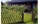The homeowner plans to build a fence along the sides and back of the property. How many feet of fencing are required to build the fence? (1 metre = 3.2808399 feet)Lots: 14w x 36.6d (m)
• Juila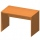Juila has a rectangular table that is 3 1/2 feet long and 1 3/4 feet wide. What is the area of Juila's table?Calculate the radius of the circle whose length is 107 cm larger than its diameter